Author: Oscar Cronquist Article last updated on October 05, 2018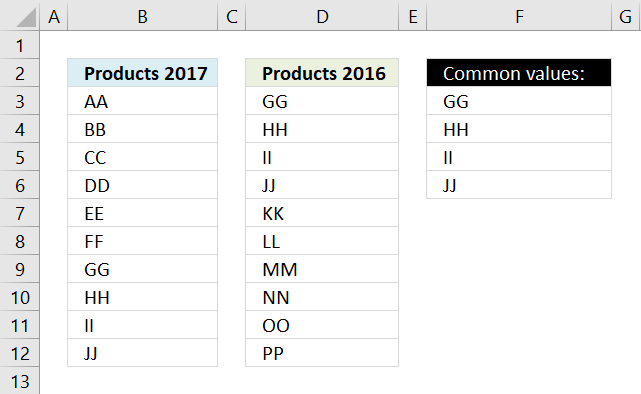Question: How can I compare two columns to find values that exists in both cell ranges?

The picture above shows two lists, one in column B and one in column D. The array formula in cell F3 extracts values that both lists have.

Array formula in cell F3:

=INDEX(\$B\$3:\$B\$12, SMALL(IF(COUNTIF(\$D\$3:\$D\$12, \$B\$3:\$B\$12), MATCH(ROW(\$B\$3:\$B\$12),ROW(\$B\$3:\$B\$12)), ""), ROWS(\$A\$1:A1)))

In this case GG, HH, II and JJ is in both lists, see picture below.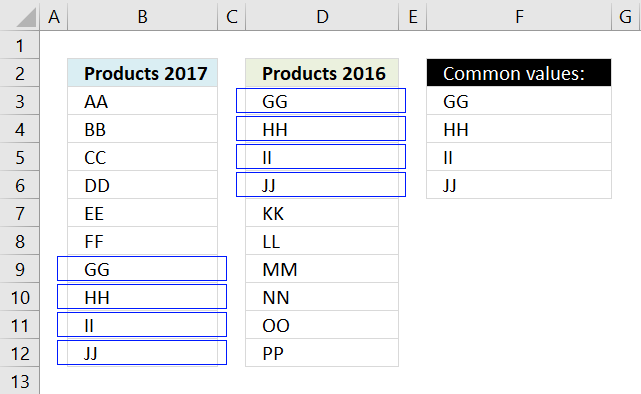The formula above can only compare two columns, however, the lists don't have to be the same size.

If you need to compare two different multicolumn cell ranges, read the following article:

Filter common values between two ranges

The image above shows an array formula in cell B12 that extracts values shared by cell range B2:D4 (One) and […]

### How to create an array formula

1. Select cell F3
2. Click in formula bar3. Copy and paste the array formula above to formula bar
4. Press and hold Ctrl + Shift simulateously
5. Press Enter
6. Release all keys

You can check using the formula bar that you did above steps right, excel tells you if a cell contains an array formula by surrounding the formula with a beginning and ending curly brackets, like this: {=array_formula}.

Don't enter these characters yourself they show up automatically if you did above steps correctly.

How to enter an array formula

Array formulas allows you to do advanced calculations not possible with regular formulas.

### How to copy array formula

Copy cell F3 and paste it to cells below as far as needed.

### Explaining array formula in cell C2

You can easily examine a formula (or array formula) that you don't understand, select the cell containing the formula. Go to tab "Formulas", click on "Evaluate Formula".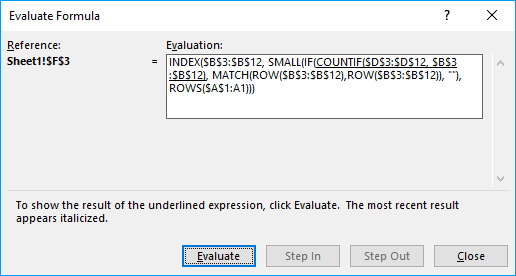The "Evaluate" button above lets you see the next "calculation" step.

#### Step 1 - Compare cell range 1 with cell range 2

The COUNTIF function lets you compare values if you enter it as an array formula and use multiple values as criteria. COUNTIF(range, criteria)

COUNTIF(\$D\$3:\$D\$12, \$B\$3:\$B\$12)

becomes

COUNTIF({"GG"; "HH"; "II"; "JJ"; "KK"; "LL"; "MM"; "NN"; "OO"; "PP"}, {"AA"; "BB"; "CC"; "DD"; "EE"; "FF"; "GG"; "HH"; "II"; "JJ"})

and returns

{0; 0; 0; 0; 0; 0; 1; 1; 1; 1}

The array is shown in column H below.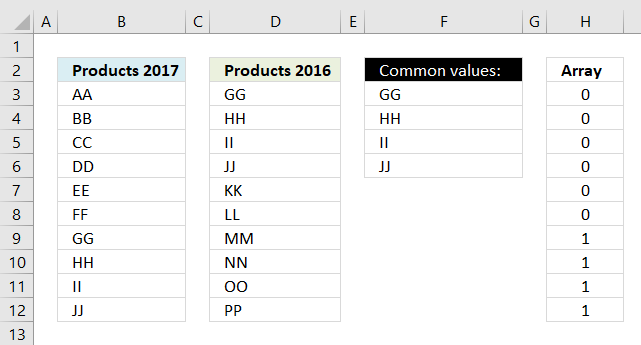This tells us that AA exists 0 (zero) times in cell range D3:D12,
BB - 0, CC - 0, DD - 0, EE-0, FF - 0

but GG is found once in cell range D3:D12 and so are HH, II, JJ.

How to use the COUNTIF function

Counts the number of cells that meet a specific condition.

#### Step 2 - Check if value exists, if so return corresponding position in array

IF({0; 0; 0; 0; 0; 0; 1; 1; 1; 1}, MATCH(ROW(\$B\$3:\$B\$12),ROW(\$B\$3:\$B\$12)), "")

becomes

IF({0; 0; 0; 0; 0; 0; 1; 1; 1; 1}, {1; 2; 3; 4; 5; 6; 7; 8; 9; 10}, "")

and returns

{""; ""; ""; ""; ""; ""; 7; 8; 9; 10}

The array is shown in column H below.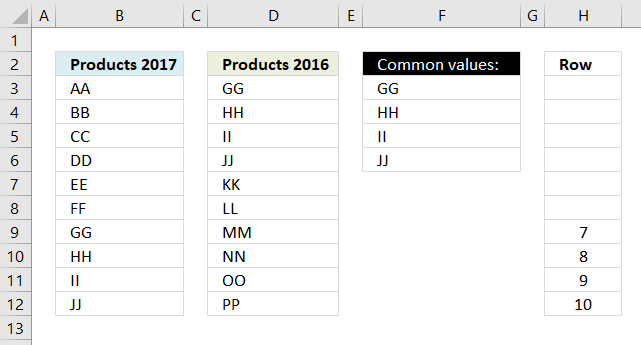How to use the IF function

Checks if a logical expression is met. Returns a specific value if TRUE and another specific value if FALSE.

#### Step 3 - Extract k-th smallest value

Until now we have been working with an array of values but excel allows us to only display one value per cell (That is not entirely true, as of Excel 2016 you can display all values in an array in one cell)

To extract a specific number from an array I use the SMALL function.

SMALL(IF(COUNTIF(\$D\$3:\$D\$12, \$B\$3:\$B\$12), MATCH(ROW(\$B\$3:\$B\$12),ROW(\$B\$3:\$B\$12)), ""), ROWS(\$A\$1:A1))

becomes

SMALL({""; ""; ""; ""; ""; ""; 7; 8; 9; 10}, ROWS(\$A\$1:A1))

becomes

SMALL({""; ""; ""; ""; ""; ""; 7; 8; 9; 10}, 1)

and returns number 7, SMALL function ignores blanks and letters.

How to use the SMALL function

The SMALL function lets you extract a number in a cell range based on how small it is compared to the other numbers in the group.

#### Step 4 - Return corresponding value

INDEX(\$B\$3:\$B\$12, SMALL(IF(COUNTIF(\$D\$3:\$D\$12, \$B\$3:\$B\$12), MATCH(ROW(\$B\$3:\$B\$12),ROW(\$B\$3:\$B\$12)), ""), ROWS(\$A\$1:A1)))

becomes

INDEX(\$B\$3:\$B\$12, 7)

becomes

INDEX({"AA"; "BB"; "CC"; "DD"; "EE"; "FF"; "GG"; "HH"; "II"; "JJ"}, 7)

and returns GG in cell F3.When you copy cell F3 and paste it to cell F4 the relative cell references changes. ROWS(\$A\$1:A1) becomes ROWS(\$A\$1:A2) and returns 2 in cell F4.

The second smallest value is then extracted from the array which is 8. The value in cell range B3:B12 in row 8 is HH. HH is returned the value returned to F4.

How to use the INDEX function

Gets a value in a specific cell range based on a row and column number.

### Download excel sample file for this tutorial

common-values1.xlsx
(Excel 2007 Workbook *.xlsx and later versions)